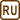## Apparent Coefficient of Thermal Expansion of Mercury

### Task number: 1807

A glass sphere with a capillary tube was filled to the brim with mercury of the volume 400 cm3 at temperature of 0 °C. In a heat bath with temperature of 100 °C, 6.228 cm3 of mercury overflowed. Determine the apparent coefficient of volume expansion of mercury.

• #### Hint – what is the apparent coefficient of expansion

The apparent coefficient of volume expansion is dependent on the container in which the liquid is placed. It states a change in the volume of the liquid in relation to the volume of the container, which, of course, also changes with temperature.

• #### Analysis

First, we must realize the difference between a real and apparent coefficient of volume expansion of liquid. While the real coefficient indicates the change of volume of liquid with increasing or decreasing temperature, the apparent coefficient refers to the container in which the liquid is placed and whose volume also changes with temperature. We can visualize this problem in such a way that there is a scale on the container which indicates the volume of the liquid inside the container, and when the container “stretches”, the scale of the container also “stretches”; therefore the scale does not show the real, but rather the apparent volume of the liquid.

From the fact that we know how much mercury spilled from the container when it was heated to the temperature of 100 °C, we can determine the apparent coefficient of expansion for both the container and liquid. We start from a known relationship for the thermal volume expansion of liquids where we know all the variables except the unknown coefficient. We can evaluate and calculate this coefficient from this equation.

• #### Given values

 t0 = 0 °C initial temperature V0 = 400 cm3 initial volume of mercury t1 = 100 °C temperature of bath ΔV = 6.228 cm3 amount of spilled mercury βa = ? apparent coefficient of expansion of mercury
• #### Solution

When solving this task, we need to realize the difference between the real and apparent coefficient of expansion of a liquid, which is described in the section Analysis. The apparent coefficient βa of a liquid and a container is therefore given by the relation

$\mathrm{\Delta}V_{\mathrm{dif}}=\beta_{\mathrm{a}} V_0(t_1-t_0),$

where V0is the initial volume of liquid, t1 − t0 is the temperature difference and ΔVdif indicates the difference between the volume change of the liquid and the volume change of the container in which the liquid is placed. In this task, the difference of volume changes ΔVdif určen právě objemem rtuti ΔV, is determined by the volume of mercury ΔV, which spilled out of the container. Therefore, we can write

$\mathrm{\Delta}V=\beta_{\mathrm{a}} V_0\left(t_1-t_0\right)$

and from here we can evaluate βa

$\beta_{\mathrm{z}}=\frac{\mathrm{\Delta}V}{V_0\left(t_1-t_0\right)}\,.$

Numerical solution

Volumes ΔV and V0 can be substituted in cm3, because β is determined by the proportion of the two volumes and therefore we can then reduce the fraction.

$\beta_{\mathrm{a}}=\frac{\mathrm{\Delta}V}{V_0\left(t_1-t_0\right)}=\frac{6.228}{400\left(100-0\right)}\,{\ ^{\circ}\mathrm{C}^{-1}}=156\cdot{10^{-6}}{\ ^{\circ}\mathrm{C}^{-1}}$

The apparent coefficient of volume expansion of mercury for our container is 156·10−6 °C−1.

• #### Apparent coefficient of expansion

The apparent coefficient of mercury in an iron container can also be calculated from the volume expansion of the two materials.

The change of the volume of mercury is:

$\mathrm{\Delta}V_{\mathrm{m}}=\beta_{\mathrm{m}} V_0\left(t_1-t_0\right),$

where βm is the coefficient of volume expansion of mercury, V0 is the volume of mercury at initial temperature and t1 − t0 is the temperature difference.

The internal volume of the container changes with the same coefficient of expansion as the volume of the material from which the container is made. We can visualize this in such a way that we tightly place an iron object into the iron container so that it completely fits. If we warm up the container with the object inside, the container and the object must expand in the same manner (they are made of the same material); the object therefore still completely fits. This means that the inner volume of the container changes with the same coefficient of expansion as the material from which the container is made.

The change in the internal volume of the container Vc is therefore given by:

$\mathrm{\Delta}V_{\mathrm{c}}=\beta_{\mathrm{c}} V_0\left(t_1-t_0\right),$

where βc is the coefficient of volumetric expansion of the material of the container, V0 is the internal volume of the container at an initial temperature and t1 − t0 is the temperature difference.

At the beginning, mercury has the same volume as the container, i.e. V0. When we subtract the equations for the volume changes, we obtain a relationship for the apparent coefficient:

$\mathrm{\Delta}V_{\mathrm{m}}-\mathrm{\Delta}V_{\mathrm{c}}=\beta_{\mathrm{m}} V_0\left(t_1-t_0\right)-\beta_{\mathrm{c}} V_0(t_1-t_0),$ $\mathrm{\Delta}V_{\mathrm{m}}-\mathrm{\Delta}V_{\mathrm{c}}=\left(\beta_{\mathrm{m}}-\beta_{\mathrm{c}}\right) V_0\left(t_1-t_0\right).$

The left side of the equation tells us how much mercury overflowed the container and on the right side there is a difference of the two expansion coefficients instead of one coefficient. This difference is the unknown apparent coefficient of mercury βa.

We deduced that:

$\beta_{\mathrm{a}}=\beta_{\mathrm{m}}-\beta_{\mathrm{c}}.$

If we substitute table values:

βm = 2·10−4 °C−1

βc = 36·10−6 °C−1

we obtain:

$\beta_{\mathrm{a}}=\beta_{\mathrm{m}}-\beta_{\mathrm{c}}=\left(2\cdot{10^{-4}}-36\cdot{10^{-6}}\right)\,\mathrm{^{\circ}C^{-1}}=1.64\cdot{10^{-4}}\,\mathrm{^{\circ}C^{-1}}.$

The table value therefore differs from the measured value by about 5 %.